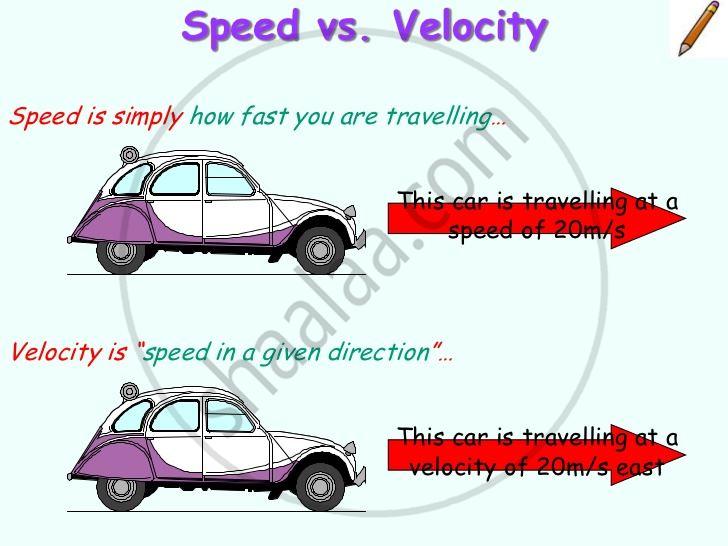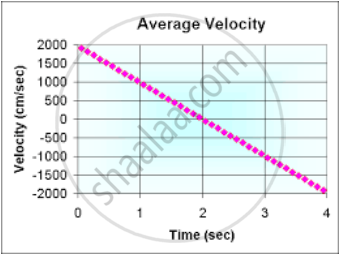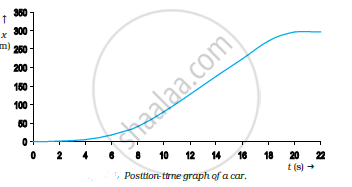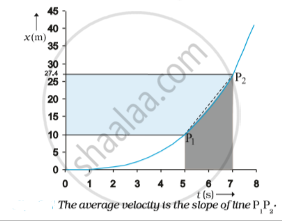# Average Velocity and Average Speed

## Notes

#### Average Velocity and Average Speed

Suppose an object is in motion, the position of that object changes with time. But how fast is the position changing with time and in what direction? To understand this, we define average velocity and average speed.Average Velocity
Average velocity is the ratio of total displacement to total time. Its direction is the same as the direction of the moving object. Even if the object is slowing down, and the magnitude of the velocity is decreasing, its direction would be still the same as the direction in which the object is moving. The magnitude of average velocity is always less than or equal to the average speed because displacement is always smaller than or equal to distance.It is a vector quantity and has units of m/s
Average velocity =Total Displacement/Total Time.
The average velocity of the particle can be positive as well as negative and its positive and negative value depends on the sign of displacement as given below. If the displacement of the particle is zero its average velocity is also zero.Consider the motion of the car from the above graph. The portion of the x-t graph between t = 0 s and t = 8 s is blown up and shown in the below fig.As seen from the plot, the average velocity of the car between time t = 5 s and t = 7s is
bar v=(x_2-x_1)/(t_2-t_1)=((27.4-10.0)m)/((7-5)s)= 8.7 ms-1

Geometrically, this is the slope of the straight line P1P2 connecting the initial position 1 P to the final position P2 as shown in the above figure.

Calculating Average Velocity
The velocity of a particle is: bar v=(d barx)/(dt)

Average Speed
The total distance travelled by the body in total time is the average speed.
Average speed = Total Distance/Total Time
It is a scalar quantity and its units are m/s.

Calculating Average Speed
Average speed is found by the first finding the total distance covered by the object and dividing it by the total time taken in travelling the distance. Example: A body covers a circle of radius 100m on 100s, implies the total distance covered by him will be d = 2πr = 200π m. So, the average speed will be v= d/t = 2π m/s

If the motion of an object is along a straight line and in the same direction, the magnitude of displacement is equal to the total path length. In that case, the magnitude of the average velocity is equal to the average speed. In case the average speed is not equal to the magnitude of the average velocity, this is because the motion involves a change in direction and so path length is greater than the magnitude of displacement. So the average speed is greater than the magnitude of the velocity.

If you would like to contribute notes or other learning material, please submit them using the button below.

### Shaalaa.com

Straight line motion Part 7 (Average and instantaneous speed) [00:10:57]
S